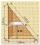# Planimetrics - math word problems

Study plane measurements, including angles, distances, and areas. In other words - measurement and calculation of shapes in the plane. Perimeter and area of plane shapes.

#### Number of problems found: 2141

• Triangular prismCalculate the volume and surface of the triangular prism ABCDEF with base of a isosceles triangle. Base's height is 16 cm, leg 10 cm, base height vc = 6 cm. The prism height is 9 cm.
• Display casePlace a glass shelf at the height of 1m from the bottom of the display case in the cabinet. How long platter will we place at this height? The display case is a rectangular triangle with 2 m and 2.5 m legs.
• ThalesThales is 1 m from the hole. The eyes are 150 cm above the ground and look into the hole with a diameter of 120 cm as shown. Calculate the depth of the hole.
• Square pyramidCalculate the pyramid's volume with the side 5cm long and with a square base, side-base has an angle of 60 degrees.
• SatinSanusha buys a piece of satin 2.4 m wide. The diagonal length of the fabric is 4m. What is the length of the piece of satin?
• Hexagon cut pyramidCalculate the volume of a regular 6-sided cut pyramid if the bottom edge is 30 cm, the top edge is 12 cm, and the side edge length is 41 cm.
• Rhombus EFGHConstruct the rhombus EFGH where e = 6.7cm, height to side h: vh = 5cm
• Diameters of circlesHow many percent of the area of a larger circle is a smaller circle if the smaller circle has a diameter 120 mm and a larger one has a diameter 300 mm?
• Diamond diagonalsCalculate the diamond's diagonal lengths if its content is 156 cm2 and the side length is 13 cm.
• Cutting circlesFrom the square 1 m side we have to cut the circles with a radius of 10 cm. How many discs we cut and how many percent will be waste?
• PagansElena cut out same circle-shaped pagans and put them on a rectangular sheet so that the neighboring pagans were touching each other and the pagans were touching the walls of the sheet on the edges. Each pagans occupied 28.26 cm2 of the bottom of the sheet
• FlowerbedIn the park there is a large circular flowerbed with a diameter of 12 m. Jakub circulated him ten times and the smaller Vojtoseven times. How many meters each went by and how many meters did Jakub run more than Vojta?
• DiameterWhat is the inside diameter of the cylinder container and if half a liter of water reaches a height 15 cm?
• Angle of deviationThe surface of the rotating cone is 30 cm2 (with circle base), its surface area is 20 cm2. Calculate the deviation of the side of this cone from the plane of the base.
• Hexagonal prismThe base of the prism is a regular hexagon consisting of six triangles with side a = 12 cm and height va = 10.4 cm. The prism height is 5 cm. Find the volume and surface of the prism.
• Children poolThe bottom of the children's pool is a regular hexagon with a = 60 cm side. The distance of opposing sides is 104 cm, the height of the pool is 45 cm. A) How many liters of water can fit into the pool? B) The pool is made of a double layer of plastic film
• The tankThe tank has 1320 liters of water. The tank has the shape of a prism, its base is an rectangle with sides a = 0,6 m and b = 1,5 m. How high does the water level reach in the tank?
• Nice prismCalculate the cuboid's surface if the sum of its edges is a + b + c = 19 cm and the body diagonal size u = 13 cm.
• Parallelogram +ľ| AB | = 76cm, | BC | = 44cm, angle BAD = 30 °. Find the area of the parallelogram.
• Juice boxThe juice box has a volume of 200ml with its base is an isosceles triangle with sides a = 4,5cm and a height of 3,4cm. How tall is the box?

Do you have an interesting mathematical word problem that you can't solve it? Submit a math problem, and we can try to solve it.

We will send a solution to your e-mail address. Solved examples are also published here. Please enter the e-mail correctly and check whether you don't have a full mailbox.

Please do not submit problems from current active competitions such as Mathematical Olympiad, correspondence seminars etc...

See also more information on Wikipedia.